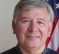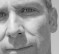## Calculate Directivity index of nonlinear line arrayStarted by 6 years ago6 replieslatest reply 6 years ago351 views

Hi

I want to calculate directivity index about nonlinear line array(curved array such that arc form).

I applied to formula https://www.usna.edu/Users/physics/ejtuchol/docume... using matlab.

but, I cannot get result what i want.

How to calculate Directivity index ?

[ - ]A few preliminaries:

1) How accurate does this calculation have to be to meet your needs?  Less accurate if the noise field is directional/unknown.  Maybe your array will reject more than you think and maybe less....

2) Can you estimate the beam pattern well enough to get an accurate enough value?

3) The receive DI is the comparison of noise received by the array output (beamformer output really) to that received by an omnidirectional receiver.  So, if  you can estimate each one then you have it.

The link you provided gives a lot of insight.  The expression for DI ends up being an inverse function of the integral of the beam pattern.  If you integrate the beam pattern over the main lobe then you have most, and probably enough, of the solution.

It's easy enough to compute the beam pattern from basic principles if you know the element placement and ignore everything else.  It's pretty much the Fourier Transform that calculates the integral (from spatial array locations in xyz to angular values).  Then you might ask yourself if the beam pattern is going to be somehow different in any significant way from what you've calculated (and why).  You didn't mention any boundary conditions or shading so this much should be a good start.

Then, given a computed beam pattern, what is the integral over the main lobe?

Yes, there's some math involved, but these are the principles.

[ - ]Interesting problem.  You first have to find the beam pattern.  This will be a function of the spacing, the curve and the wavelength.  That will be a hard problem because it will be a function of both $$\theta$$ and $$\phi$$ unless your detectors are on a sphere.  This seems like something a person could earn a Ph.D. or two on.

If your detectors are lying on the ocean floor, computing the DI would be a nightmare.  It'd be a lot simpler to just measure it from a bunch of angles and wavelengths and interpolate what you need.

The formulas I've found online won't work, they assume the detectors are in a plane relative to the incoming wave.  Your beam pattern is different, so you need to start from scratch.  It will be challenging, if not impossible to do analytically.

Patience, persistence, truth,

Dr. mike

[ - ]OK.  I'm not a sonar guy, so maybe I'm vastly oversimplifying this.

But shouldn't you be able to find the gain of an array of transponders just by adding up the gains of each transponder, taking into account both the delay caused by the direction of the external wave and the delay that you're intentionally imposing from within?

I'm sure I'm not using the right nomenclature here, but for reception:

$$r_a (t) = \sum_i A_i s (t - \tau_{i,i} - \tau_{e,i})$$

where $$s (t)$$ is the signal that's transmitted at the source, $$A_i$$ is the gain of the $$i^{th}$$ transponder for sound from the direction to the source, $$\tau_{i,i}$$ is the internal delay you're adding to get the directivity you want, and $$\tau_{e,i}$$ is the delay imposed by the speed of sound, the geometry of the array, and the direction of arrival of the sound wave.

So all you do is sum up the contribution of each transponder for each given angle (and perhaps frequency, if bandwidth is a concern), and get an answer. Do that for lots and lots of angles and you should have an antenna pattern.

This seems like simple physics.  What am I missing?

[ - ]Hi Mike,

to inline Mathjax, you can use the

$$...$$

I need to document this better.  Thanks for participating to the DSPRelated forum Mike.

[ - ]